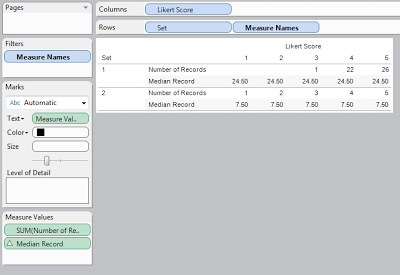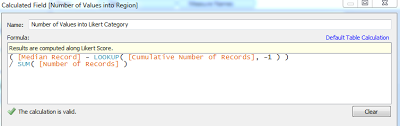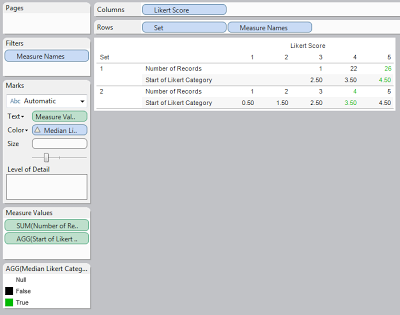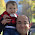## Tuesday, March 5, 2013

### Calculating the Median of a Likert (Survey) Scale in Tableau

Today, we will look at how to calculate the "median" of Likert Scale in Tableau.  Median is in quotes because this is not a true median, it is more like a weighted average.  However, I believe it gives you far more information than a true median and, therefore, is worth pursuing.  For those of you who are not familiar with a Likert Scale, it is as follows:

1 - Strongly Disagree
2 - Disagree
3 - Neither Agree nor Disagree
4 - Agree
5 - Strongly Agree

This is the common 5-point version; however, there are adaptations of 6 and 7 points as well.  On the bright side, the algorithm in this post will work for any number of points with no alterations.  For the first time, I had to create my own data set for this post.  The sets are as follows:Sample Data
This idea is courtesy of a poster on the Tableau Forums, Stuart Ramsbottom. (If he gives me a better way to credit him, I will add it here.)  His original post on the Tableau forums can be found HERE.  Set 1 is actually his set of data, while Set 2 is a set I made up to test the algorithm.

Step 1:
• The median record is found as a traditional median, i.e. Total Number of Records / 2.
• Create the following calculated fieldMedian RecordMedian Record Test
Step 2:
• Find the upper threshold for each Likert Category, i.e. Calculate the Cumulative Number of Records.
• Create the following calculated fieldCumulative Number of RecordsCumulative Number of Records Test
Step 3:
• Using these upper thresholds, find the Likert Category corresponding the Median Record, i.e. < Threshold( Likert Category - 1 ) < Median Record <= Threshold( Likert Category ).
To me, this seems to be a difficult concept to communicate.  To put it more simply, you must find the Likert Category that the Median Record falls in.

• Create the following calculated fieldMedian Likert Category
• Find how "far" into the Likert Category the Median Record is, i.e. (Median Record - Lower Threshold of Likert Category) / Number of Records in this Likert Category
• Create the following calculated fieldNumber of Values into Likert CategoryNumber of Values into Likert Category Test
Step 5:
• Find the starting value of the Likert Category, i.e. the average of Likert Category and Likert Category - 1 = Likert Category - .5
• Create the following calculated fieldStart of Likert CategoryStart of Likert Category Test
Step 6:
• Take the Starting Value of the Median Likert Category, add the Number of Values into the Likert Category, i.e. Starting Value of Category + Number of Values into Category.
• Apply this calculation only to the Median Likert Category (It is incorrect for every other category)
• Create the following calculated fieldMedian Likert Score
Now, we can use see the final result of our work, the "Median" Likert Score for our data.Final Result
We hope you found this informative.  Thanks for reading.

Associate Consultant
Mariner, LLC
llewellyn.wb@gmail.com

1.2.1.